Calculus Volume 1

# 4.3Maxima and Minima

Calculus Volume 14.3 Maxima and Minima

### Learning Objectives

• 4.3.1 Define absolute extrema.
• 4.3.2 Define local extrema.
• 4.3.3 Explain how to find the critical points of a function over a closed interval.
• 4.3.4 Describe how to use critical points to locate absolute extrema over a closed interval.

Given a particular function, we are often interested in determining the largest and smallest values of the function. This information is important in creating accurate graphs. Finding the maximum and minimum values of a function also has practical significance because we can use this method to solve optimization problems, such as maximizing profit, minimizing the amount of material used in manufacturing an aluminum can, or finding the maximum height a rocket can reach. In this section, we look at how to use derivatives to find the largest and smallest values for a function.

### Absolute Extrema

Consider the function $f(x)=x2+1f(x)=x2+1$ over the interval $(−∞,∞).(−∞,∞).$ As $x→±∞,x→±∞,$ $f(x)→∞.f(x)→∞.$ Therefore, the function does not have a largest value. However, since $x2+1≥1x2+1≥1$ for all real numbers $xx$ and $x2+1=1x2+1=1$ when $x=0,x=0,$ the function has a smallest value, 1, when $x=0.x=0.$ We say that 1 is the absolute minimum of $f(x)=x2+1f(x)=x2+1$ and it occurs at $x=0.x=0.$ We say that $f(x)=x2+1f(x)=x2+1$ does not have an absolute maximum (see the following figure).

Figure 4.12 The given function has an absolute minimum of 1 at $x=0.x=0.$ The function does not have an absolute maximum.

### Definition

Let $ff$ be a function defined over an interval $II$ and let $c∈I.c∈I.$ We say $ff$ has an absolute maximum on $II$ at $cc$ if $f(c)≥f(x)f(c)≥f(x)$ for all $x∈I.x∈I.$ We say $ff$ has an absolute minimum on $II$ at $cc$ if $f(c)≤f(x)f(c)≤f(x)$ for all $x∈I.x∈I.$ If $ff$ has an absolute maximum on $II$ at $cc$ or an absolute minimum on $II$ at $c,c,$ we say $ff$ has an absolute extremum on $II$ at $c.c.$

Before proceeding, let’s note two important issues regarding this definition. First, the term absolute here does not refer to absolute value. An absolute extremum may be positive, negative, or zero. Second, if a function $ff$ has an absolute extremum over an interval $II$ at $c,c,$ the absolute extremum is $f(c).f(c).$ The real number $cc$ is a point in the domain at which the absolute extremum occurs. For example, consider the function $f(x)=1/(x2+1)f(x)=1/(x2+1)$ over the interval $(−∞,∞).(−∞,∞).$ Since

$f(0)=1≥1x2+1=f(x)f(0)=1≥1x2+1=f(x)$

for all real numbers $x,x,$ we say $ff$ has an absolute maximum over $(−∞,∞)(−∞,∞)$ at $x=0.x=0.$ The absolute maximum is $f(0)=1.f(0)=1.$ It occurs at $x=0,x=0,$ as shown in Figure 4.13(b).

A function may have both an absolute maximum and an absolute minimum, just one extremum, or neither. Figure 4.13 shows several functions and some of the different possibilities regarding absolute extrema. However, the following theorem, called the Extreme Value Theorem, guarantees that a continuous function $ff$ over a closed, bounded interval $[a,b][a,b]$ has both an absolute maximum and an absolute minimum.

Figure 4.13 Graphs (a), (b), and (c) show several possibilities for absolute extrema for functions with a domain of $(−∞,∞).(−∞,∞).$ Graphs (d), (e), and (f) show several possibilities for absolute extrema for functions with a domain that is a bounded interval.

### Theorem4.1

#### Extreme Value Theorem

If $ff$ is a continuous function over the closed, bounded interval $[a,b],[a,b],$ then there is a point in $[a,b][a,b]$ at which $ff$ has an absolute maximum over $[a,b][a,b]$ and there is a point in $[a,b][a,b]$ at which $ff$ has an absolute minimum over $[a,b].[a,b].$

The proof of the extreme value theorem is beyond the scope of this text. Typically, it is proved in a course on real analysis. There are a couple of key points to note about the statement of this theorem. For the extreme value theorem to apply, the function must be continuous over a closed, bounded interval. If the interval $II$ is open or the function has even one point of discontinuity, the function may not have an absolute maximum or absolute minimum over $I.I.$ For example, consider the functions shown in Figure 4.13(d), (e), and (f). All three of these functions are defined over bounded intervals. However, the function in graph (e) is the only one that has both an absolute maximum and an absolute minimum over its domain. The extreme value theorem cannot be applied to the functions in graphs (d) and (f) because neither of these functions is continuous over a closed, bounded interval. Although the function in graph (d) is defined over the closed interval $[0,4],[0,4],$ the function is discontinuous at $x=2.x=2.$ The function has an absolute maximum over $[0,4][0,4]$ but does not have an absolute minimum. The function in graph (f) is continuous over the half-open interval $[0,2),[0,2),$ but is not defined at $x=2,x=2,$ and therefore is not continuous over a closed, bounded interval. The function has an absolute minimum over $[0,2),[0,2),$ but does not have an absolute maximum over $[0,2).[0,2).$ These two graphs illustrate why a function over a bounded interval may fail to have an absolute maximum and/or absolute minimum.

Before looking at how to find absolute extrema, let’s examine the related concept of local extrema. This idea is useful in determining where absolute extrema occur.

### Local Extrema and Critical Points

Consider the function $ff$ shown in Figure 4.14. The graph can be described as two mountains with a valley in the middle. The absolute maximum value of the function occurs at the higher peak, at $x=2.x=2.$ However, $x=0x=0$ is also a point of interest. Although $f(0)f(0)$ is not the largest value of $f,f,$ the value $f(0)f(0)$ is larger than $f(x)f(x)$ for all $xx$ near 0. We say $ff$ has a local maximum at $x=0.x=0.$ Similarly, the function $ff$ does not have an absolute minimum, but it does have a local minimum at $x=1x=1$ because $f(1)f(1)$ is less than $f(x)f(x)$ for $xx$ near 1.

Figure 4.14 This function $ff$ has two local maxima and one local minimum. The local maximum at $x=2x=2$ is also the absolute maximum.

### Definition

A function $ff$ has a local maximum at $cc$ if there exists an open interval $II$ containing $cc$ such that $II$ is contained in the domain of $ff$ and $f(c)≥f(x)f(c)≥f(x)$ for all $x∈I.x∈I.$ A function $ff$ has a local minimum at $cc$ if there exists an open interval $II$ containing $cc$ such that $II$ is contained in the domain of $ff$ and $f(c)≤f(x)f(c)≤f(x)$ for all $x∈I.x∈I.$ A function $ff$ has a local extremum at $cc$ if $ff$ has a local maximum at $cc$ or $ff$ has a local minimum at $c.c.$

Note that if $ff$ has an absolute extremum at $cc$ and $ff$ is defined over an interval containing $c,c,$ then $f(c)f(c)$ is also considered a local extremum. If an absolute extremum for a function $ff$ occurs at an endpoint, we do not consider that to be a local extremum, but instead refer to that as an endpoint extremum.

Given the graph of a function $f,f,$ it is sometimes easy to see where a local maximum or local minimum occurs. However, it is not always easy to see, since the interesting features on the graph of a function may not be visible because they occur at a very small scale. Also, we may not have a graph of the function. In these cases, how can we use a formula for a function to determine where these extrema occur?

To answer this question, let’s look at Figure 4.14 again. The local extrema occur at $x=0,x=0,$ $x=1,x=1,$ and $x=2.x=2.$ Notice that at $x=0x=0$ and $x=1,x=1,$ the derivative $f′(x)=0.f′(x)=0.$ At $x=2,x=2,$ the derivative $f′(x)f′(x)$ does not exist, since the function $ff$ has a corner there. In fact, if $ff$ has a local extremum at a point $x=c,x=c,$ the derivative $f′(c)f′(c)$ must satisfy one of the following conditions: either $f′(c)=0f′(c)=0$ or $f′(c)f′(c)$ is undefined. Such a value $cc$ is known as a critical point and it is important in finding extreme values for functions.

### Definition

Let $cc$ be an interior point in the domain of $f.f.$ We say that $cc$ is a critical number of $ff$ if $f′(c)=0f′(c)=0$ or $f′(c)f′(c)$ is undefined. We call the point $(c, f(c))(c, f(c))$ a critical point of $ff$. Note that these two terms are often used interchangeably in this text and elsewhere.

As mentioned earlier, if $ff$ has a local extremum at a point $x=c,x=c,$ then $cc$ must be a critical point of $f.f.$ This fact is known as Fermat’s theorem.

### Theorem4.2

#### Fermat’s Theorem

If $ff$ has a local extremum at $cc$ and $ff$ is differentiable at $c,c,$ then $f′(c)=0.f′(c)=0.$

#### Proof

Suppose $ff$ has a local extremum at $cc$ and $ff$ is differentiable at $c.c.$ We need to show that $f′(c)=0.f′(c)=0.$ To do this, we will show that $f′(c)≥0f′(c)≥0$ and $f′(c)≤0,f′(c)≤0,$ and therefore $f′(c)=0.f′(c)=0.$ Since $ff$ has a local extremum at $c,c,$ $ff$ has a local maximum or local minimum at $c.c.$ Suppose $ff$ has a local maximum at $c.c.$ The case in which $ff$ has a local minimum at $cc$ can be handled similarly. There then exists an open interval $II$ such that $f(c)≥f(x)f(c)≥f(x)$ for all $x∈I.x∈I.$ Since $ff$ is differentiable at $c,c,$ from the definition of the derivative, we know that

$f′(c)=limx→cf(x)−f(c)x−c.f′(c)=limx→cf(x)−f(c)x−c.$

Since this limit exists, both one-sided limits also exist and equal $f′(c).f′(c).$ Therefore,

$f′(c)=limx→c+f(x)−f(c)x−c,f′(c)=limx→c+f(x)−f(c)x−c,$
(4.4)

and

$f′(c)=limx→c−f(x)−f(c)x−c.f′(c)=limx→c−f(x)−f(c)x−c.$
(4.5)

Since $f(c)f(c)$ is a local maximum, we see that $f(x)−f(c)≤0f(x)−f(c)≤0$ for $xx$ near $c.c.$ Therefore, for $xx$ near $c,c,$ but $x>c,x>c,$ we have $f(x)−f(c)x−c≤0.f(x)−f(c)x−c≤0.$ From Equation 4.4 we conclude that $f′(c)≤0.f′(c)≤0.$ Similarly, it can be shown that $f′(c)≥0.f′(c)≥0.$ Therefore, $f′(c)=0.f′(c)=0.$

From Fermat’s theorem, we conclude that if $ff$ has a local extremum at $c,c,$ then either $f′(c)=0f′(c)=0$ or $f′(c)f′(c)$ is undefined. In other words, local extrema can only occur at critical points.

Note this theorem does not claim that a function $ff$ must have a local extremum at a critical point. Rather, it states that critical points are candidates for local extrema. For example, consider the function $f(x)=x3.f(x)=x3.$ We have $f′(x)=3x2=0f′(x)=3x2=0$ when $x=0.x=0.$ Therefore, $x=0x=0$ is a critical point. However, $f(x)=x3f(x)=x3$ is increasing over $(−∞,∞),(−∞,∞),$ and thus $ff$ does not have a local extremum at $x=0.x=0.$ In Figure 4.15, we see several different possibilities for critical points. In some of these cases, the functions have local extrema at critical points, whereas in other cases the functions do not. Note that these graphs do not show all possibilities for the behavior of a function at a critical point.

Figure 4.15 (a–e) A function $ff$ has a critical point at $cc$ if $f′(c)=0f′(c)=0$ or $f′(c)f′(c)$ is undefined. A function may or may not have a local extremum at a critical point.

Later in this chapter we look at analytical methods for determining whether a function actually has a local extremum at a critical point. For now, let’s turn our attention to finding critical points. We will use graphical observations to determine whether a critical point is associated with a local extremum.

### Example 4.12

#### Locating Critical Points

For each of the following functions, find all critical points. Use a graphing utility to determine whether the function has a local extremum at each of the critical points.

1. $f(x)=13x3−52x2+4xf(x)=13x3−52x2+4x$
2. $f(x)=(x2−1)3f(x)=(x2−1)3$
3. $f(x)=4x1+x2f(x)=4x1+x2$

### Checkpoint4.12

Find all critical points for $f(x)=x3−12x2−2x+1.f(x)=x3−12x2−2x+1.$

### Locating Absolute Extrema

The extreme value theorem states that a continuous function over a closed, bounded interval has an absolute maximum and an absolute minimum. As shown in Figure 4.13, one or both of these absolute extrema could occur at an endpoint. If an absolute extremum does not occur at an endpoint, however, it must occur at an interior point, in which case the absolute extremum is a local extremum. Therefore, by Fermat’s Theorem, the point $cc$ at which the local extremum occurs must be a critical point. We summarize this result in the following theorem.

### Theorem4.3

#### Location of Absolute Extrema

Let $ff$ be a continuous function over a closed, bounded interval $I.I.$ The absolute maximum of $ff$ over $II$ and the absolute minimum of $ff$ over $II$ must occur at endpoints of $II$ or at critical points of $ff$ in $I.I.$

With this idea in mind, let’s examine a procedure for locating absolute extrema.

### Problem-Solving Strategy

#### Problem-Solving Strategy: Locating Absolute Extrema over a Closed Interval

Consider a continuous function $ff$ defined over the closed interval $[a,b].[a,b].$

1. Evaluate $ff$ at the endpoints $x=ax=a$ and $x=b.x=b.$
2. Find all critical points of $ff$ that lie over the interval $(a,b)(a,b)$ and evaluate $ff$ at those critical points.
3. Compare all values found in (1) and (2). From Location of Absolute Extrema, the absolute extrema must occur at endpoints or critical points. Therefore, the largest of these values is the absolute maximum of $f.f.$ The smallest of these values is the absolute minimum of $f.f.$

Now let’s look at how to use this strategy to find the absolute maximum and absolute minimum values for continuous functions.

### Example 4.13

#### Locating Absolute Extrema

For each of the following functions, find the absolute maximum and absolute minimum over the specified interval and state where those values occur.

1. $f(x)=−x2+3x−2f(x)=−x2+3x−2$ over $[1,3].[1,3].$
2. $f(x)=x2−3x2/3f(x)=x2−3x2/3$ over $[0,2].[0,2].$

### Checkpoint4.13

Find the absolute maximum and absolute minimum of $f(x)=x2−4x+3f(x)=x2−4x+3$ over the interval $[1,4].[1,4].$

At this point, we know how to locate absolute extrema for continuous functions over closed intervals. We have also defined local extrema and determined that if a function $ff$ has a local extremum at a point $c,c,$ then $cc$ must be a critical point of $f.f.$ However, $cc$ being a critical point is not a sufficient condition for $ff$ to have a local extremum at $c.c.$ Later in this chapter, we show how to determine whether a function actually has a local extremum at a critical point. First, however, we need to introduce the Mean Value Theorem, which will help as we analyze the behavior of the graph of a function.

### Section 4.3 Exercises

90.

In precalculus, you learned a formula for the position of the maximum or minimum of a quadratic equation $y=ax2+bx+c,y=ax2+bx+c,$ which was $h=−b(2a).h=−b(2a).$ Prove this formula using calculus.

91.

If you are finding an absolute minimum over an interval $[a,b],[a,b],$ why do you need to check the endpoints? Draw a graph that supports your hypothesis.

92.

If you are examining a function over an interval $(a,b),(a,b),$ for $aa$ and $bb$ finite, is it possible not to have an absolute maximum or absolute minimum?

93.

When you are checking for critical points, explain why you also need to determine points where $f'(x)f'(x)$ is undefined. Draw a graph to support your explanation.

94.

Can you have a finite absolute maximum for $y=ax2+bx+cy=ax2+bx+c$ over $(−∞,∞)?(−∞,∞)?$ Explain why or why not using graphical arguments.

95.

Can you have a finite absolute maximum for $y=ax3+bx2+cx+dy=ax3+bx2+cx+d$ over $(−∞,∞)(−∞,∞)$ assuming a is non-zero? Explain why or why not using graphical arguments.

96.

Let $mm$ be the number of local minima and $MM$ be the number of local maxima. Can you create a function where $M>m+2?M>m+2?$ Draw a graph to support your explanation.

97.

Is it possible to have more than one absolute maximum? Use a graphical argument to prove your hypothesis.

98.

Is it possible to have no absolute minimum or maximum for a function? If so, construct such a function. If not, explain why this is not possible.

99.

[T] Graph the function $y=eax.y=eax.$ For which values of $a,a,$ on any infinite domain, will you have an absolute minimum and absolute maximum?

For the following exercises, determine where the local and absolute maxima and minima occur on the graph given. Assume the graph represents the entirety of each function. For any extrema located at an endpoint, approximate the x-value.

100.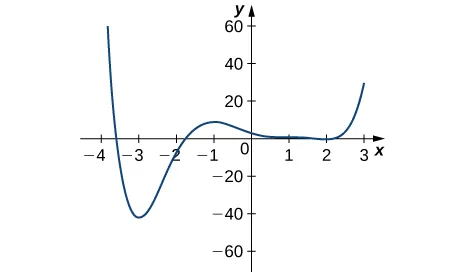101.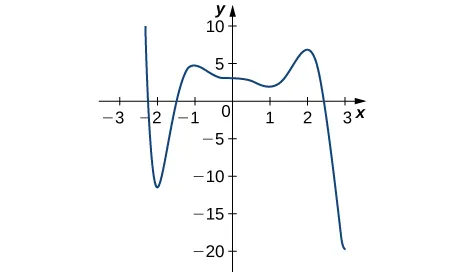102.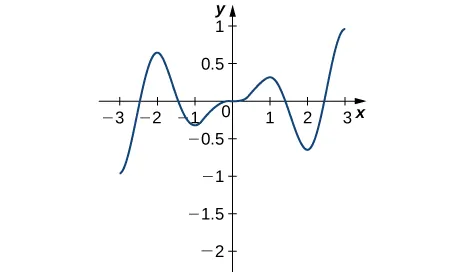103.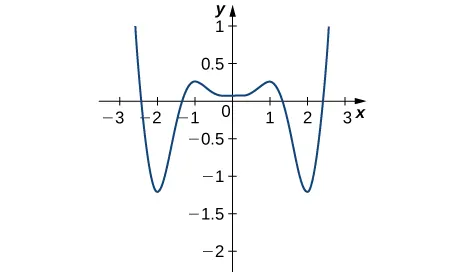For the following problems, draw graphs of $f(x),f(x),$ which is continuous, over the interval $[−4,4][−4,4]$ with the following properties:

104.

Absolute maximum at $x=2x=2$ and absolute minima at $x=±3x=±3$

105.

Absolute minimum at $x=1x=1$ and absolute maximum at $x=2x=2$

106.

Absolute maximum at $x=4,x=4,$ absolute minimum at $x=−1,x=−1,$ local maximum at $x=−2,x=−2,$ and a critical point that is not a maximum or minimum at $x=2x=2$

107.

Absolute maxima at $x=2x=2$ and $x=−3,x=−3,$ local minimum at $x=1,x=1,$ and absolute minimum at $x=4x=4$

For the following exercises, find the critical points in the domains of the following functions.

108.

$y = 4 x 3 − 3 x y = 4 x 3 − 3 x$

109.

$y = 4 x − x 2 y = 4 x − x 2$

110.

$y = 1 x − 1 y = 1 x − 1$

111.

$y = ln ( x − 2 ) y = ln ( x − 2 )$

112.

$y = tan ( x ) y = tan ( x )$

113.

$y = 4 − x 2 y = 4 − x 2$

114.

$y = x 3 / 2 − 3 x 5 / 2 y = x 3 / 2 − 3 x 5 / 2$

115.

$y = x 2 − 1 x 2 + 2 x − 3 y = x 2 − 1 x 2 + 2 x − 3$

116.

$y = sin 2 ( x ) y = sin 2 ( x )$

117.

$y = x + 1 x y = x + 1 x$

For the following exercises, find the local and/or absolute extrema for the functions over the specified domain.

118.

$f(x)=x2+3f(x)=x2+3$ over $[−1,4][−1,4]$

119.

$y=x2+2xy=x2+2x$ over $[1,4][1,4]$

120.

$y=(x−x2)2y=(x−x2)2$ over $[−1,1][−1,1]$

121.

$y=1(x−x2)y=1(x−x2)$ over $(0,1)(0,1)$

122.

$y=9−xy=9−x$ over $[1,9][1,9]$

123.

$y=x+sin(x)y=x+sin(x)$ over $[0,2π][0,2π]$

124.

$y=x1+xy=x1+x$ over $[0,100][0,100]$

125.

$y=|x+1|+|x−1|y=|x+1|+|x−1|$ over $[−3,2][−3,2]$

126.

$y=x−x3y=x−x3$ over $[0,4][0,4]$

127.

$y=sinx+cosxy=sinx+cosx$ over $[0,2π][0,2π]$

128.

$y=4sinθ−3cosθy=4sinθ−3cosθ$ over $[0,2π][0,2π]$

For the following exercises, find the local and absolute minima and maxima for the functions over $(−∞,∞).(−∞,∞).$

129.

$y = x 2 + 4 x + 5 y = x 2 + 4 x + 5$

130.

$y = x 3 − 12 x y = x 3 − 12 x$

131.

$y = 3 x 4 + 8 x 3 − 18 x 2 y = 3 x 4 + 8 x 3 − 18 x 2$

132.

$y = x 3 ( 1 − x ) 6 y = x 3 ( 1 − x ) 6$

133.

$y = x 2 + x + 6 x − 1 y = x 2 + x + 6 x − 1$

134.

$y = x 2 − 1 x − 1 y = x 2 − 1 x − 1$

For the following functions, use a calculator to graph the function and to estimate the absolute and local maxima and minima. Then, solve for them explicitly.

135.

[T] $y=3x1−x2y=3x1−x2$

136.

[T] $y=x+sin(x)y=x+sin(x)$

137.

[T] $y=12x5+45x4+20x3−90x2−120x+3y=12x5+45x4+20x3−90x2−120x+3$

138.

[T] $y=x3+6x2−x−30x−2y=x3+6x2−x−30x−2$

139.

[T] $y=4−x24+x2y=4−x24+x2$

140.

A company that produces cell phones has a cost function of $C=x2−1200x+36,400,C=x2−1200x+36,400,$ where $CC$ is cost in dollars and $xx$ is number of cell phones produced (in thousands). How many units of cell phone (in thousands) minimizes this cost function?

141.

A ball is thrown into the air and its position is given by $h(t)=−4.9t2+60t+5m.h(t)=−4.9t2+60t+5m.$ Find the height at which the ball stops ascending. How long after it is thrown does this happen?

For the following exercises, consider the production of gold during the California gold rush (1848–1888). The production of gold can be modeled by $G(t)=(25t)(t2+16),G(t)=(25t)(t2+16),$ where $tt$ is the number of years since the rush began $(0≤t≤40)(0≤t≤40)$ and $GG$ is ounces of gold produced (in millions). A summary of the data is shown in the following figure.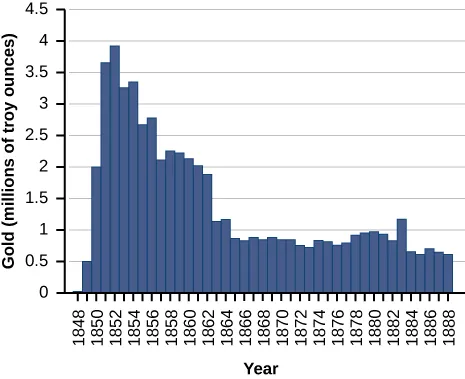142.

Find when the maximum (local and absolute) gold production occurred, and the amount of gold produced during that maximum.

143.

Find when the minimum (local and absolute) gold production occurred. What was the amount of gold produced during this minimum?

Find the critical points, maxima, and minima for the following piecewise functions.

144.

$y = { x 2 − 4 x 0 ≤ x ≤ 1 x 2 − 4 1 < x ≤ 2 y = { x 2 − 4 x 0 ≤ x ≤ 1 x 2 − 4 1 < x ≤ 2$

145.

$y = { x 2 + 1 x ≤ 1 x 2 − 4 x + 5 x > 1 y = { x 2 + 1 x ≤ 1 x 2 − 4 x + 5 x > 1$

For the following exercises, find the critical points of the following generic functions. Are they maxima, minima, or neither? State the necessary conditions.

146.

$y=ax2+bx+c,y=ax2+bx+c,$ given that $a>0a>0$

147.

$y=(x−1)a,y=(x−1)a,$ given that $a>1a>1$ and a is an integer.

Order a print copy

As an Amazon Associate we earn from qualifying purchases.